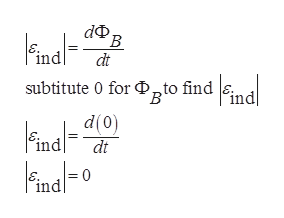Question
228 views

29.14     A flat rectangular coil of dimensions l and w is pulled with uniform speed v through a uniform magnetic field B with the plane of its area perpendicular to the field (Fig. E29.14).

(a) Find the emf induced in this coil.

(b) If the speed and magnetic field are both tripled, what is the induced emf?

check_circle

Step 1

a)

Formula to calculate the magnetic flux is, where B and A are perpendicular to each other

Step 2

Formula to calculate the induced emf is,help_outlineImage TranscriptionclosedФ, В ind dt subtitute 0 for Dto find d Find0 ind (0)p \Fnd fullscreen
Step 3

Thus the coil placed in the uniform magnetic field which produced a constant magnetic flux. Hence the emf...

### Want to see the full answer?

See Solution

#### Want to see this answer and more?

Solutions are written by subject experts who are available 24/7. Questions are typically answered within 1 hour.*

See Solution
*Response times may vary by subject and question.
Tagged in

### Electric Charges and Fields